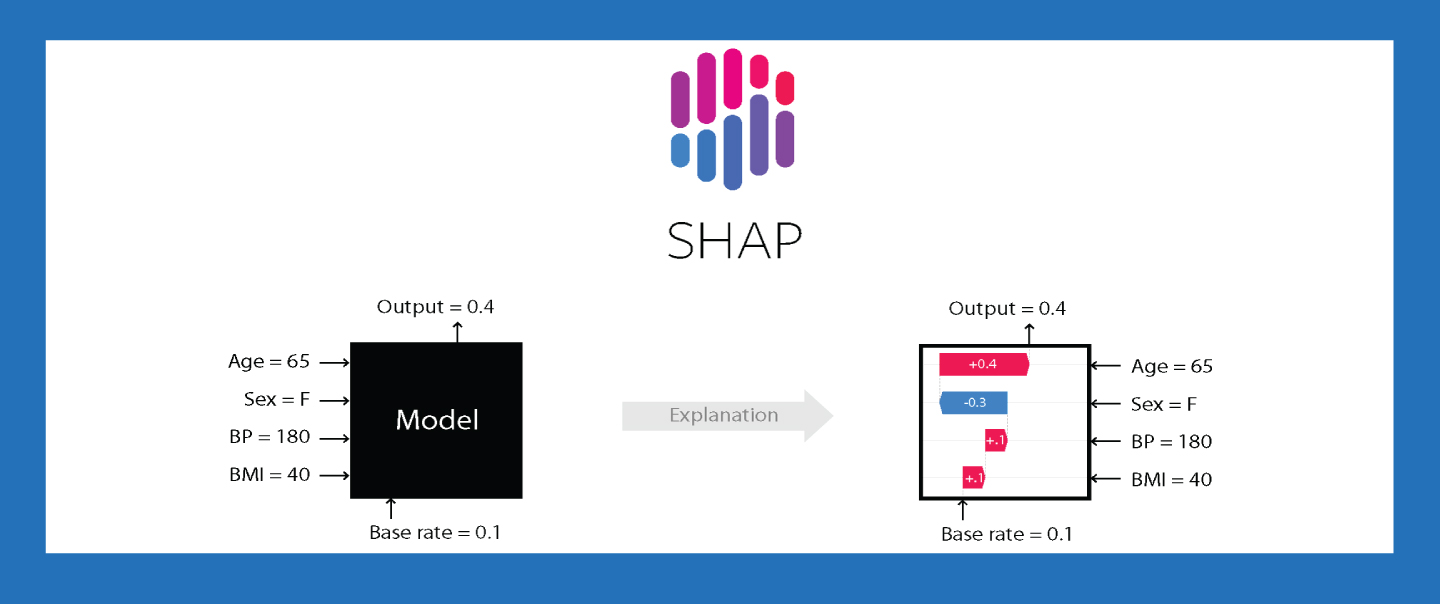## Model Explainability Using SHAP

Machine learning models are frequently viewed as "black boxes", which makes it challenging to analyse them. We require Explainable Machine Learning algorithms that reveal some of these qualities in order to comprehend what are the key features that influence the model's output.

Machine learning interpretability becomes an element of the criterion for a decent model when investments are at stake and when you're working with real-world issues. You may estimate the contribution of each feature in your data to the prediction of the model using feature importance. It helps to determine which features are having the biggest impact on your model's decision-making by conducting feature importance tests. As a result, you can take action by eliminating features that have little bearing on the model's predictions and concentrating on enhancing the more important qualities. This has a big impact on model performance.

The SHAP (Shapely Additive Explanations) approach is one of these methods, which explains how each feature influences the model and enables local and global analysis for the given dataset and issue.

## SHAP implementation:

We must train our model before utilizing SHAP. Take a dataset and train any model such as a CatBoost Regressor. We must make an Explainer object and use it to assess a sample or the entire dataset in order to calculate SHAP values for the model. For each feature in our model, we will have one SHAP value for each observation.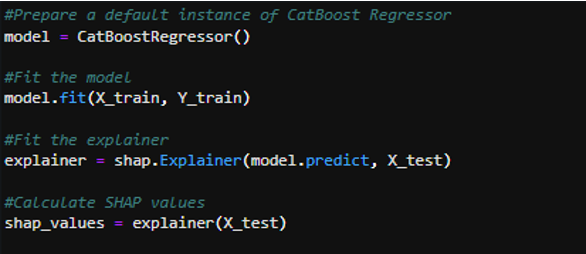## SHAP Plots:

The SHAP values can be seen using some great tools in the Shap library. Let’s see some of the plots below.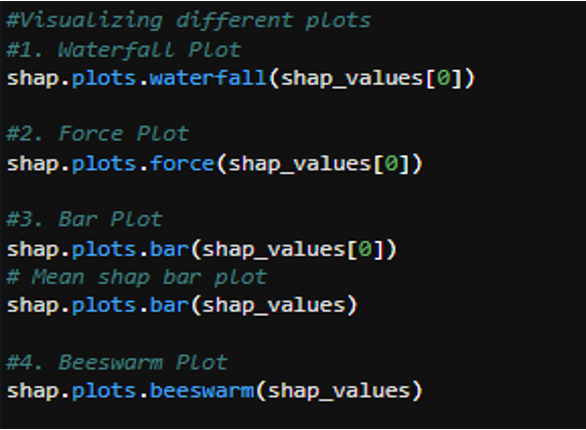1. Waterfall plot: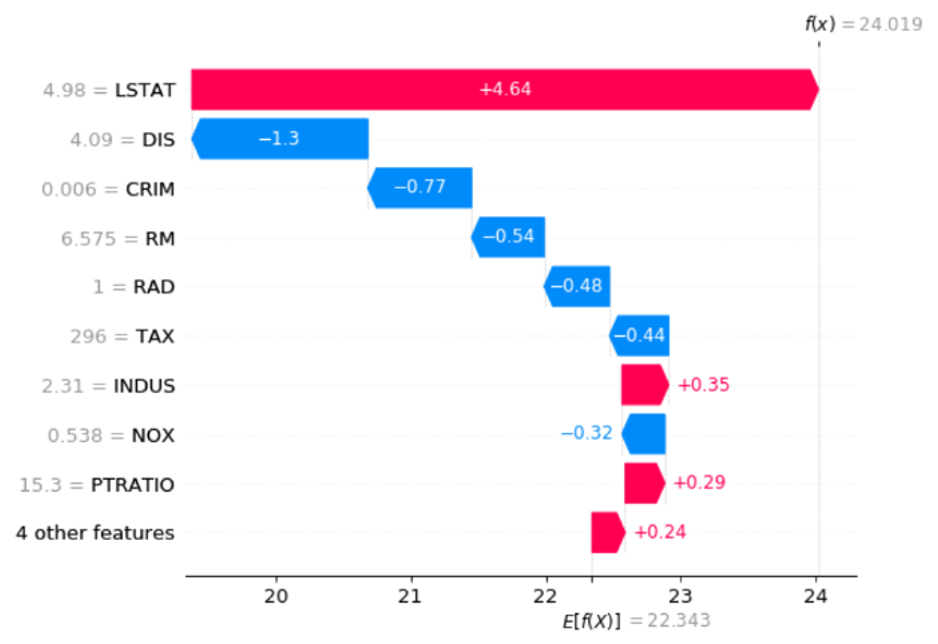Waterfall plot (Source: image by the author)

The colour of each arrow in this plot indicates whether a particular factor has a positive (red) or negative (blue) impact on the prediction. The absolute SHAP value of each arrow's related property determines its length. Over the arrow, the SHAP value is written, and on the vertical axis, the value of the relevant feature is also written (for example in the plot above, the value of the feature LSTAT is 4.98, and its SHAP value is 4.64). The arrow is red and points to the right if the SHAP value is positive. When the SHAP value is negative, it is blue and moves to the left. Finally, by following these arrows, we arrive at the model prediction value f(x)=24.019. The feature with the highest absolute SHAP value is placed at the top because the features are ordered by their absolute SHAP values.

2. Force Plot: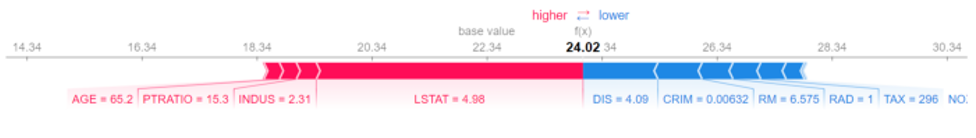Force Plot (source : image by the author)

Similar to a waterfall plot, a force plot has stacked horizontal arrows instead. According to how long they are, the red and blue arrows are arranged so that the red arrows grow longer from left to right and the blue arrows grow longer from right to left. Behind each matching arrow is put the value of the related feature, not its SHAP value.

3. Bar Plot: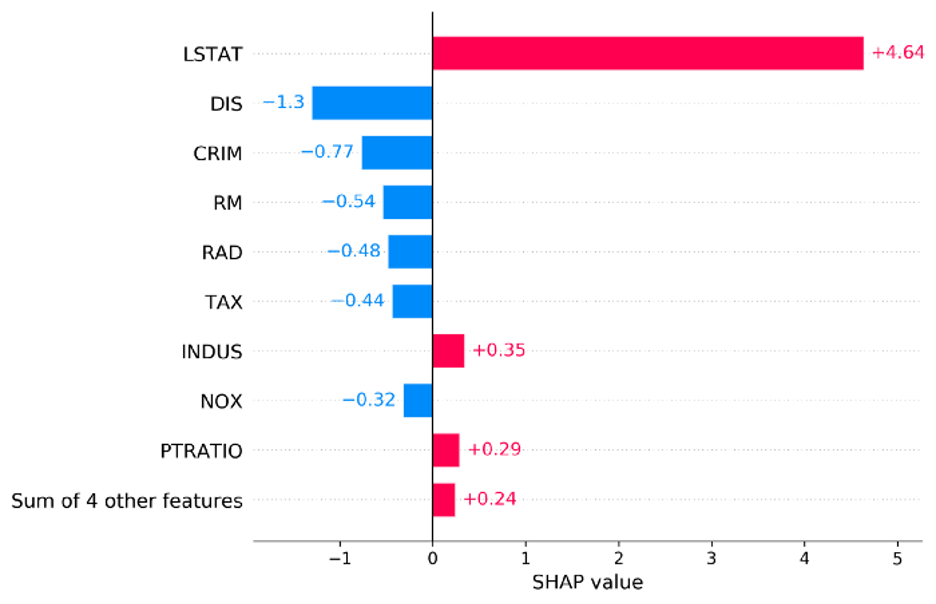Bar Plot (Source: Image by the author)

The SHAP values can also be shown using a bar plot. Each bar's length reflects the absolute SHAP value of the relevant feature. The SHAP values are written beside each bar and the bar is coloured red/blue for positive/negative SHAP values. The features are arranged in ascending order by their absolute SHAP values.

With a bar plot, we may use several instances as well. Then it will average the absolute SHAP values across all instances and order the features accordingly. Alongside the bars is also displayed the average of the absolute SHAP values.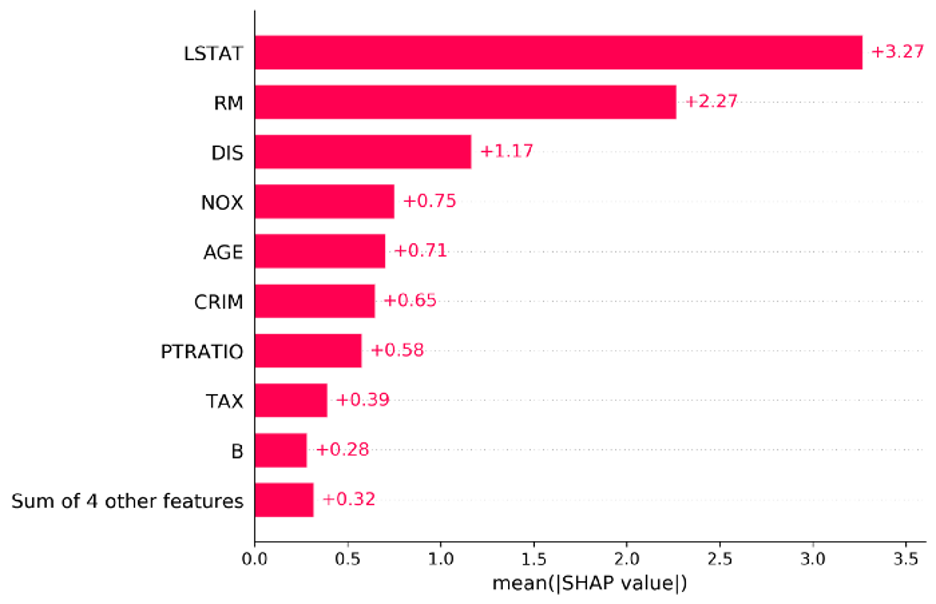Mean Shap: (Source: Image by the author)

4. Beeswarm Plot: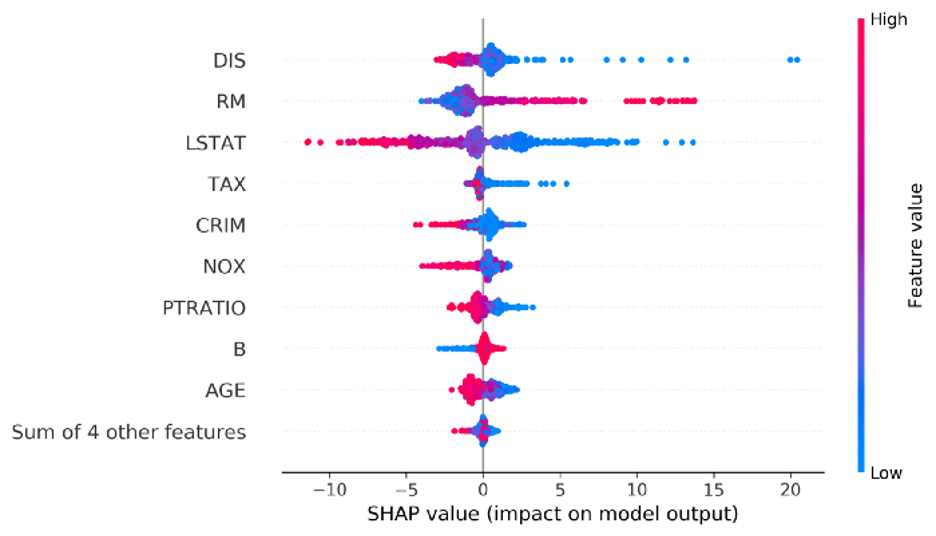Beeswarm Plot (Source: Image by the author)

The Beeswarm plot resembles a bar plot, but instead of bars, there are dots for each SHAP value. Each dot's x location indicates the SHAP value for the related feature. The value of the appropriate attribute determines the colour of the dots. The instance with the greater value for each feature is red, whereas the other instance is blue. The features are arranged according to the mean SHAP values.

In this article, we have reviewed the application of SHAP library and its popular plots. The plots of SHAP could help user visually understand the feature importance and influence. So SHAP is a useful tool to explain and visualize machine learning which was normally "black box".